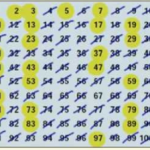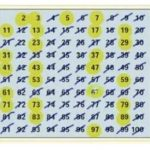Smartick is an advanced online program that teaches kids math and coding in only 15 min. a day

# I want to learn about: Divisibility

Content about divisibility in elementary mathematics. Here you will find information about divisibility adapted for elementary aged children to make learning mathematics easier and more fun.

Dec30

## Prime Numbers and Composite NumbersToday we are going to learn about prime numbers and composite numbers. What is a prime number? A prime number is a number (greater than 1) that is only divisible by 1 and itself. What are composite numbers? They are numbers (greater than 1) that are not prime. They are divisible by 1, by itself […]

Dec26

## Greatest Common Factor (GCF)

In this post we are going to learn what the Greatest Common Factor (GCF) is and how to calculate it. What is the Greatest Common Factor (GCF)?  It is the biggest number that divides exactly into two or more numbers. Terms: Factor: A factor of a number is a number that divides evenly into the […]